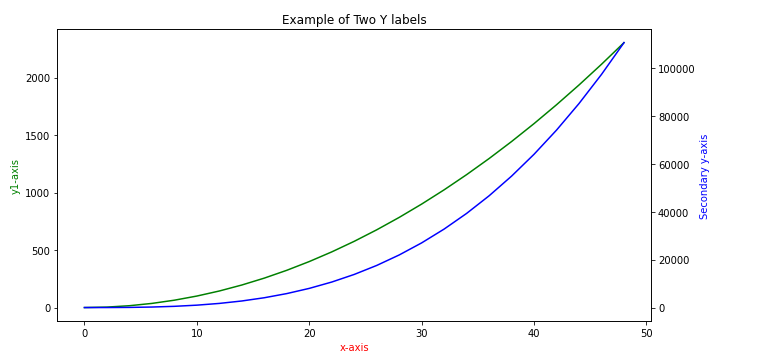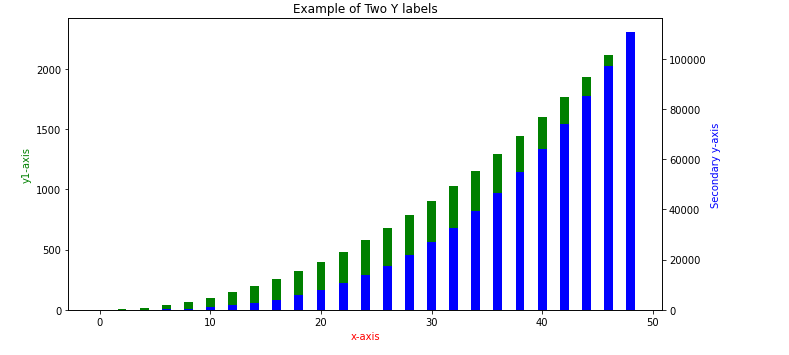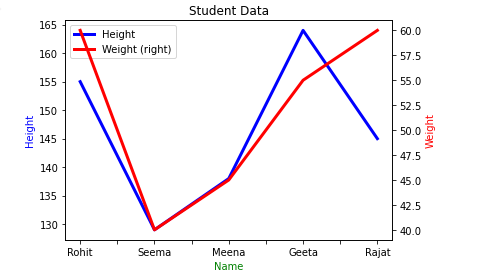# How to Add a Y-Axis Label to the Secondary Y-Axis in Matplotlib?

• Last Updated : 04 Aug, 2022

Sometimes while analyzing any data through graphs we need two x or y-axis to get some more insights into the data. Matplotlib library of Python is the most popular data visualization library, and we can generate any type of plot in Matplotlib. We can create a plot that has two y-axes and can provide different labels to both of the y-axis. We can make a plot with two different y-axes by creating or using two different axes objects with the help of twinx() function. First, we create figure and axis objects and make the first plot. And we also set the x and y-axis labels with the help of the axis object created.

Axes object: Axes are the most basic and flexible unit for creating subplots. Axes are used for the placement of plots at any location in the figure. A given figure of the plot can contain many axes, but a given axes object can only be in one figure of the plot.

### Approach:

• Import packages.
• Use the axes object and create a subplot.
• Using the twinx() define the plot values.
• Now label the axis.
• Show plot.

Example 1:

In this example we have created a plot with two different y-axes by using two different axes objects a and a2 with the help of twinx() function. ax.twinx() creates a new Axes object ax2 for a y-axis that is opposite to the original y-axis. The second axes object ax2 is used to make the plot of the second y-axis variable and to update its label.

## Python3

 `# Adding a Y-Axis Label to the Secondary Y-Axis in Matplotlib``# importing the libraries``import` `numpy as np``import` `matplotlib.pyplot as plt` `# creating data for plot``# data arrangement between 0 and 50``# with the difference of 2``# x-axis``x ``=` `np.arange(``0``, ``50``, ``2``)` `# y-axis values``y1 ``=` `x``*``*``2` `# secondary y-axis values``y2 ``=` `x``*``*``3` `# plotting figures by creating axes object``# using subplots() function``fig, ax ``=` `plt.subplots(figsize ``=` `(``10``, ``5``))``plt.title(``'Example of Two Y labels'``)` `# using the twinx() for creating another``# axes object for secondary y-Axis``ax2 ``=` `ax.twinx()``ax.plot(x, y1, color ``=` `'g'``)``ax2.plot(x, y2, color ``=` `'b'``)` `# giving labels to the axises``ax.set_xlabel(``'x-axis'``, color ``=` `'r'``)``ax.set_ylabel(``'y1-axis'``, color ``=` `'g'``)` `# secondary y-axis label``ax2.set_ylabel(``'Secondary y-axis'``, color ``=` `'b'``)` `# defining display layout``plt.tight_layout()` `# show plot``plt.show()`

Output:Example 2:

In this example we have created a bar plot using same method.

## Python3

 `# Adding a Y-Axis Label to the``# Secondary Y-Axis in Matplotlib``# importing the libraries``import` `numpy as np``import` `matplotlib.pyplot as plt` `# creating data for plot``# data arrangement between 0 and 50 with the difference of 2``# x-axis values``x ``=` `np.arange(``0``, ``50``, ``2``)` `#y-axis values``y1 ``=` `x``*``*``2` `# secondary y-axis values``y2 ``=` `x``*``*``3` `# plotting figures by creating axes object``# using subplots() function``fig, ax ``=` `plt.subplots(figsize ``=` `(``10``, ``5``))``plt.title(``'Example of Two Y labels'``)` `# using the twinx() for creating``# another axes object for secondary y-Axis``ax2 ``=` `ax.twinx()``# creating a bar plot``ax.bar(x, y1, color ``=` `'g'``)``ax2.bar(x, y2, color ``=` `'b'``)` `# giving labels to the axises``ax.set_xlabel(``'x-axis'``, color ``=` `'r'``)``ax.set_ylabel(``'y1-axis'``, color ``=` `'g'``)` `# secondary y-axis label``ax2.set_ylabel(``'Secondary y-axis'``, color ``=` `'b'``)` `# defining display layout``plt.tight_layout()` `# show plot``plt.show()`

Output:Example 3:

We can add a y-axis label to the secondary y-axis in pandas too. Generating a plot from DataFrame and also without using twinx() function. In this example, we will use simple DataFrame.plot() function with some parameters to specify the plot.

When we set the secondary_y parameter to be True in DataFrame.plot method, it returns different axes that can be used to set the labels.

## Python3

 `# Adding a Y-Axis Label to``# the Secondary Y-Axis in Matplotlib``# importing the libraries``import` `pandas as pd``import` `matplotlib.pyplot as plt` `#creating dataframe for plot``dataset ``=` `pd.DataFrame({``'Name'``:[``'Rohit'``, ``'Seema'``,``                                ``'Meena'``, ``'Geeta'``,``                                ``'Rajat'``],``                        ` `                   ``'Height'``: [``155``,``129``,``138``,``164``,``145``],``                   ``'Weight'``: [``60``,``40``,``45``,``55``,``60``]})` `# creating axes object and defining plot``ax ``=` `dataset.plot(kind ``=` `'line'``, x ``=` `'Name'``,``                  ``y ``=` `'Height'``, color ``=` `'Blue'``,``                  ``linewidth ``=` `3``)` `ax2 ``=` `dataset.plot(kind ``=` `'line'``, x ``=` `'Name'``,``                   ``y ``=` `'Weight'``, secondary_y ``=` `True``,``                   ``color ``=` `'Red'``,  linewidth ``=` `3``,``                   ``ax ``=` `ax)` `#title of the plot``plt.title(``"Student Data"``)` `#labeling x and y-axis``ax.set_xlabel(``'Name'``, color ``=` `'g'``)``ax.set_ylabel(``'Height'``, color ``=` `"b"``)``ax2.set_ylabel(``'Weight'``, color ``=` `'r'``)` `#defining display layout``plt.tight_layout()` `#show plot``plt.show()`

Output:In above example, the plot is created without using twinx() function, but we have created two axis object ax and ax2 as given in other examples for two y-axis to make the plot with two y-axis and update its label.

My Personal Notes arrow_drop_up